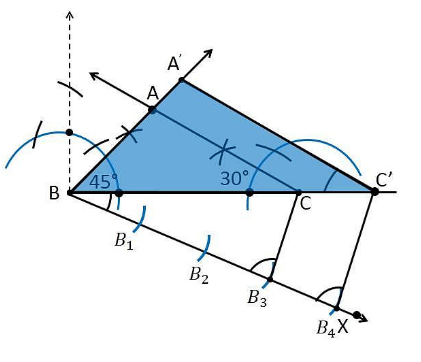Guru

# Draw a triangle ABC with side BC = 7 cm, ∠ B = 45°, ∠ A = 105°. Then, construct a triangle whose sides are 4/3 times the corresponding sides of ∆ ABC. Q.6

• 0

Sir please suggest me the best and simple way to solve the problem of question no.6 of class 10th ncert of constructions chapter of exercise 11.1, give me the best way to solve this problem Draw a triangle ABC with side BC = 7 cm, ∠ B = 45°, ∠ A = 105°. Then, construct a triangle whose sides are 4/3 times the corresponding sides of ∆ ABC.

Share

1. To find ∠C:

Given:

∠B = 45°, ∠A = 105°

We know that,

Sum of all interior angles in a triangle is 180°.

∠A+∠B +∠C = 180°

105°+45°+∠C = 180°

∠C = 180° − 150°

∠C = 30°

So, from the property of triangle, we get ∠C = 30°

Construction Procedure:

The required triangle can be drawn as follows.

1. Draw a ΔABC with side measures of base BC = 7 cm, ∠B = 45°, and ∠C = 30°.

2. Draw a ray BX makes an acute angle with BC on the opposite side of vertex A.

3. Locate 4 points (as 4 is greater in 4 and 3), such as B1, B2, B3, B4, on the ray BX.

4. Join the points B3C.

5. Draw a line through B4 parallel to B3C which intersects the extended line BC at C’.

6. Through C’, draw a line parallel to the line AC that intersects the extended line segment at C’.

7. Therefore, ΔA’BC’ is the required triangle.Justification:

The construction of the given problem can be justified by proving that

Since the scale factor is 4/3, we need to prove

A’B = (4/3)AB

BC’ = (4/3)BC

A’C’= (4/3)AC

From the construction, we get A’C’ || AC

In ΔA’BC’ and ΔABC,

∴ ∠A’C’B = ∠ACB (Corresponding angles)

∠B = ∠B (common)

∴ ΔA’BC’ ∼ ΔABC (From AA similarity criterion)

Since the corresponding sides of the similar triangle are in the same ratio, it becomes

Therefore, A’B/AB = BC’/BC= A’C’/AC

So, it becomes A’B/AB = BC’/BC= A’C’/AC = 4/3

Hence, justified.

• 0